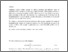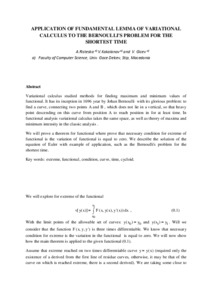# Application of fundamental lemma of variational calculus to the Bernoulli's problem for the shortest time

Risteska, Aleksandra and Kokalanov, Vasko and Gicev, Vlado (2015) Application of fundamental lemma of variational calculus to the Bernoulli's problem for the shortest time. In: ITRO 2015, 26 June 2015, Zrenjanin, Serbia.Preview Text APPLICATION+OF+FUNDAMENTAL+LEMMA+OF+VARIATIONAL+CALCULUS+TO+THE+BERNOULLI'S++PROBLEM.pdf Download (521kB) | Preview

## Abstract

Variational calculus studied methods for finding maximum and minimum values of functional. It has its inception in 1696 year by Johan Bernoulli with its glorious problem: to find a curve, connecting two points A and B , which does not lie in a vertical, so that heavy point descending on this curve from position A to reach position in for at least time. In functional analysis variational calculus takes the same space, as well as theory of maxima and minimum intensity in the classic analysis .
We will prove a theorem for functional where prove that necessary condition for extreme of functional is the variation of functional is equal to zero. We describe the solution of the equation of Euler with example of application, such as the Bernoulli's problem for the shortest time.

Item Type: Conference or Workshop Item (Paper) Natural sciences > MatematicsNatural sciences > Physical sciences Faculty of Computer Science Aleksandra Risteska 30 Sep 2015 12:41 03 Mar 2017 08:43 https://eprints.ugd.edu.mk/id/eprint/13887View Item